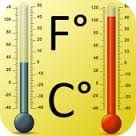Problems
323 - Temperature Conversion

Temperature Conversion

Time Limit: 1 sec

The Problem

Make a simple program that read one variable name T. Here ‘T’ denote the temperature of today in degree Fahrenheit. Print the temperature (T) in degree Celsius.The Input

Input file contain a series of line, each line contains a single integer number T (T<200). Input is terminated by EOF.

The Output

For each line of input, print the temperature in degree Celsius in a separate line.

Sample Input

50

120

157

Sample Output

10.00 C

48.89 C

69.44 C

Problem Setter: Nezam Uddin